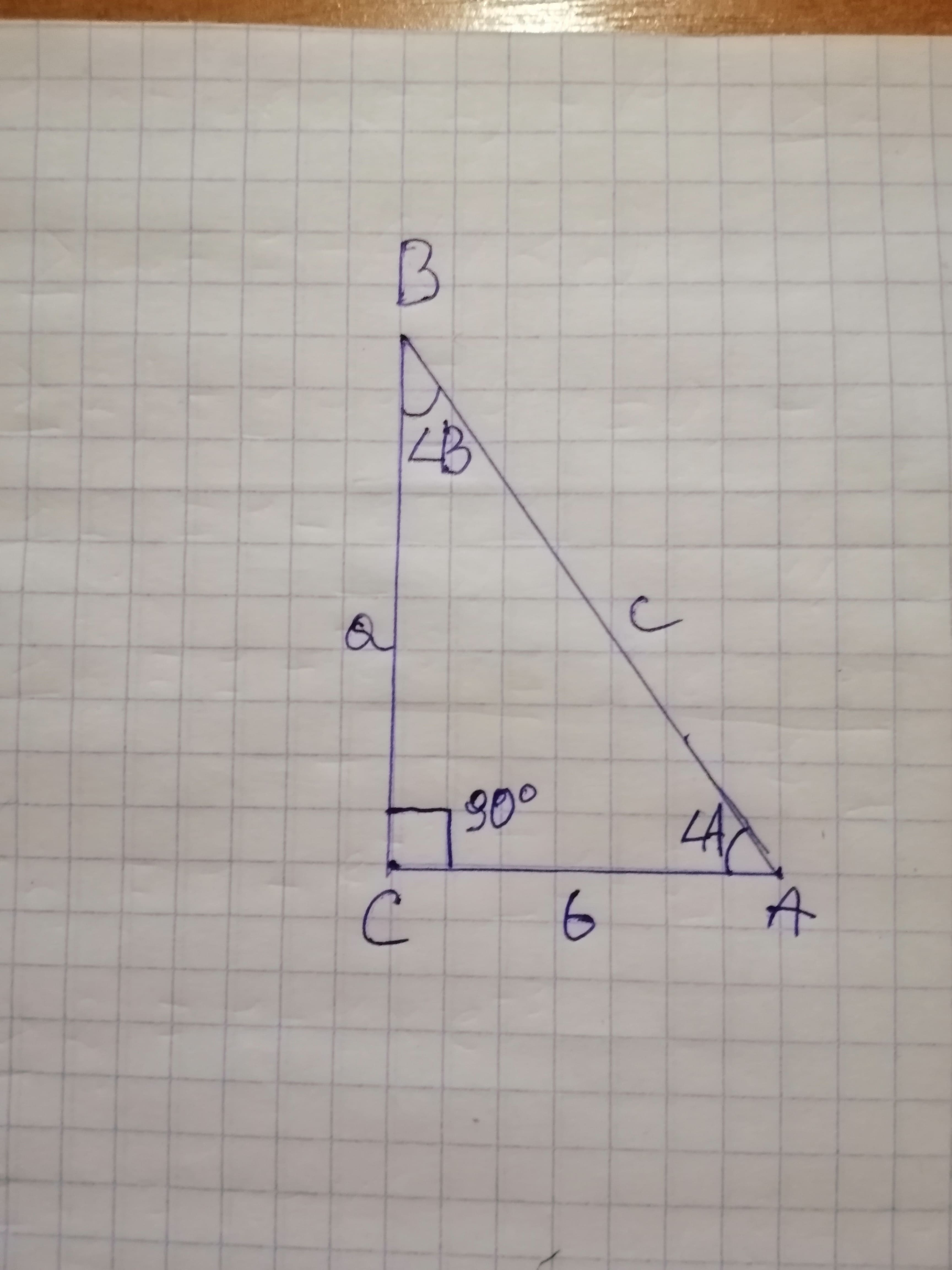# Refer to right triangle ABC in which C = 90^{circ}. Solve each triangle. a = 4.37, c = 6.21jernplate8 2021-02-15 Answered
Refer to right triangle ABC in which $C={90}^{\circ }.$ Solve each triangle.
You can still ask an expert for help

• Questions are typically answered in as fast as 30 minutes

Solve your problem for the price of one coffee

• Math expert for every subject
• Pay only if we can solve itTalisha

Data analysis
Given $\mathrm{△}ABC$ is a right angled triangle at C.
And $a=4.37$ units
$c=6.21$ units
To solve the triangle.
The triangle is as follows,Solution
Since the triangle is right angle,

$=BC/AB$
$=a/c$
$=4.37/6.21$

(Rounded to two decimals)
Sum of all angles in a triangle $={180}^{\circ }$

Since the triangle is right angle,

$=AC/AB$
$=b/c$

$=6.21\left(0.710185376\right)$
units
(Rounded to two decimals)